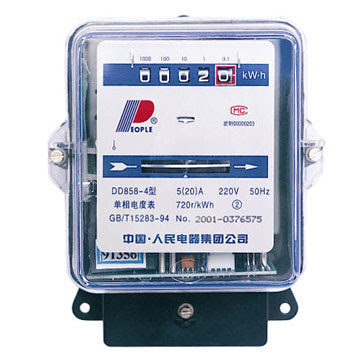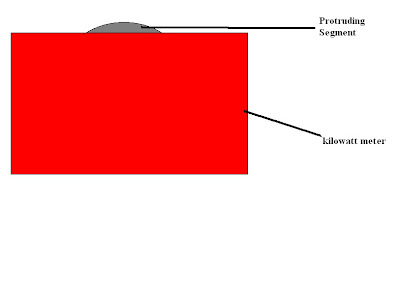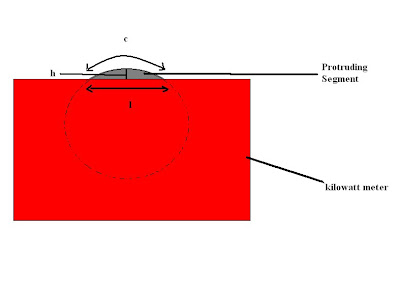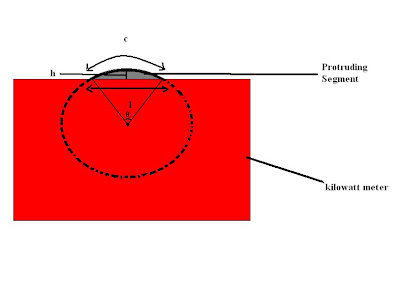## Tuesday, January 19, 2010

### Open Question

Was shopping on Sunday, and saw this thing in a shopAnd saw the metal plate spinning inside.
Suddenly a thought struck me: what is the size of that metal plate?
Given the circumference of the protruding segment, can it lead me to know the circumference of the whole plate?

So I went back and worked out the solutions.The data that I can acquire without harming(dissecting la) the meter are the h,l and c as shown belowQ: WHAT IS THE TOTAL CIRCUMFERENCE OF THE METAL PLATE?
by having those 3 data, we can deduce a relationship by using the angle θ of the segment
θ=57.296c/r______(1)

and also
c=0.01745rθ_______(2)

since we can measure c, so c is a known variable. Thus we can solve equation (1) and (2) by using simultaneous equation to get the radius of the metal plate r.

Once we have the radius then we can calculate the total surface area of the metal plate and also its circumference, assuming the plate is very very thin.
With these we can even calculate the electric potential of the plate, and how it affects the overall performance of the meter.

Anyway this is just random but I guess it's nice to just pop up with random application problem and train our brain to think =)

Malcolm